Next: Is it possible to Up: Computing the Delaunay complex Previous: Computing the Delaunay complex   Contents

### Sample session with cdd+

Consider a simple two dimensional case: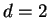,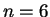and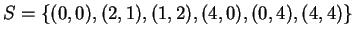. In principle the session below will work in any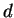and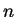, although the computation time depends heavily on the size.

The first step is to write down the system of linear inequalities in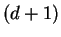variables as explained in 3.2: for each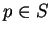,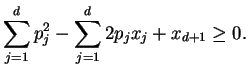For our example, we have: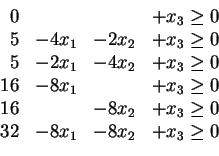We denote by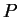the polyhedron of all solutions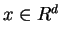satisfying the inequalities above. Now we prepare an input file of cdd+. The file must be in polyhedra format and for the system above, it is rather straightforward since it essentially codes the coefficients of the system.

* filename: vtest_vo.ine
H-representation
begin
6   4   integer
0  0  0  1
5 -4 -2  1
5 -2 -4  1
16 -8  0  1
16  0 -8  1
32 -8 -8  1
end
incidence
input_adjacency


The last two lines incidence'' and input_adjacency'' are options for cdd+. They are not necessary for listing the vertices of the polyhedron, but but can be used to generate the nearest neighbor sets and the adjacency of Voronoi cells.

Now, by running cdd+ with commands:

% cddr+ vtest.ine

or
% cddf+ vtest.ine

we obtain three files: vtest_vo.ext (all extreme points and rays), vtest_vo.iad (adjacency of facet inequalities) and vtest_vo.ecd (incidence of extreme points/rays and inequalities). Note that cddr+ runs in rational (exact) arithmetic and cddf+ runs in floating-point arithmetic. cddf+ runs much faster than cddr+ but it may not give a correct answer.

The file vtest_vo.ext would be something like the following:

*FINAL RESULT:
*Number of Vertices =6, Rays =4
begin
10  4  rational
0 -1 0 0
1 -3/2 2 0
1 5/6 5/6 0
1 2 -3/2 0
0 0 -1 0
1 27/10 27/10 56/5
1 15/4 2 14
0 1 0 8
0 0 1 8
1 2 15/4 14
end
hull


The output contains all the vertices and extreme rays of the (unbounded) polyhedronin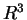. Namely each row starting with 1'' represents a vertex. So the second row

  1 -3/2 2 0

represents the vertex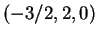. Each row starting with 0'' represents an extreme ray, e.g. the first row
  0 -1 0 0

represents the ray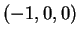.

By ignoring the last components, we obtain the set of six Voronoi vertices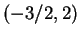,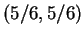,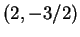,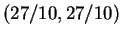,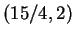and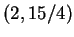and four Voronoi rays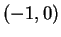,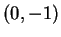,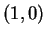and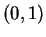.

With the option incidence, cdd+ outputs vtest_vo.ecd file:

begin
10  6  7
3 :  1 5 7
3 :  1 3 5
3 :  1 2 3
3 :  1 2 4
3 :  1 4 7
3 :  2 3 6
3 :  2 4 6
3 :  4 6 7
3 :  5 6 7
3 :  3 5 6
end


Each row corresponds to the same row in vtest_vo.ine file. For example, the second data

  3 :  1 3 5

says the second data in vtest_vo.ine file:
  1 -3/2 2 0

is a voronoi vertex whose nearest neighbor set is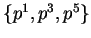. Also, this set corresponds to a Delaunay cell. Similarly, the first row
  3 :  1 5 7

indicates the ray (the first output in vtest_vo.ine file)
  0 -1 0 0

is determined by 1, 5 and 7th halfspaces. The 7th halfspace is an artificial one corresponding to the infinity. So this ray is determined by the input points 1 and 5 and going to infinity.

Thus, the index sets (triples, in this case) not containing the infinity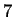determine all Delaunay cells, and those containingcorrespond to the Voronoi rays.

Finally, look at the vtest_vo.iad file:

begin
7
1 5 : 2 3 4 5 7
2 4 : 1 3 4 6
3 4 : 1 2 5 6
4 4 : 1 2 6 7
5 4 : 1 3 6 7
6 5 : 2 3 4 5 7
7 4 : 1 4 5 6
end


This file contains the graph structure of the Delaunay complex and equivalently the adjacency of Voronoi cells in the Voronoi diagram.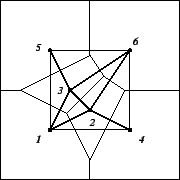The first line in this file:

 1 5 : 2 3 4 5 7

says the point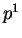is adjacent to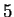neighbors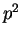,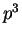,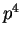,and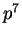. Here, the pointis the artificial infinity point which is considered adjacent to any input point whose Voronoi cell is unbounded.

As we remarked before, this graph information can be computed much more efficiently by linear programming. See 3.5.Next: Is it possible to Up: Computing the Delaunay complex Previous: Computing the Delaunay complex   Contents
Komei Fukuda 2004-08-26